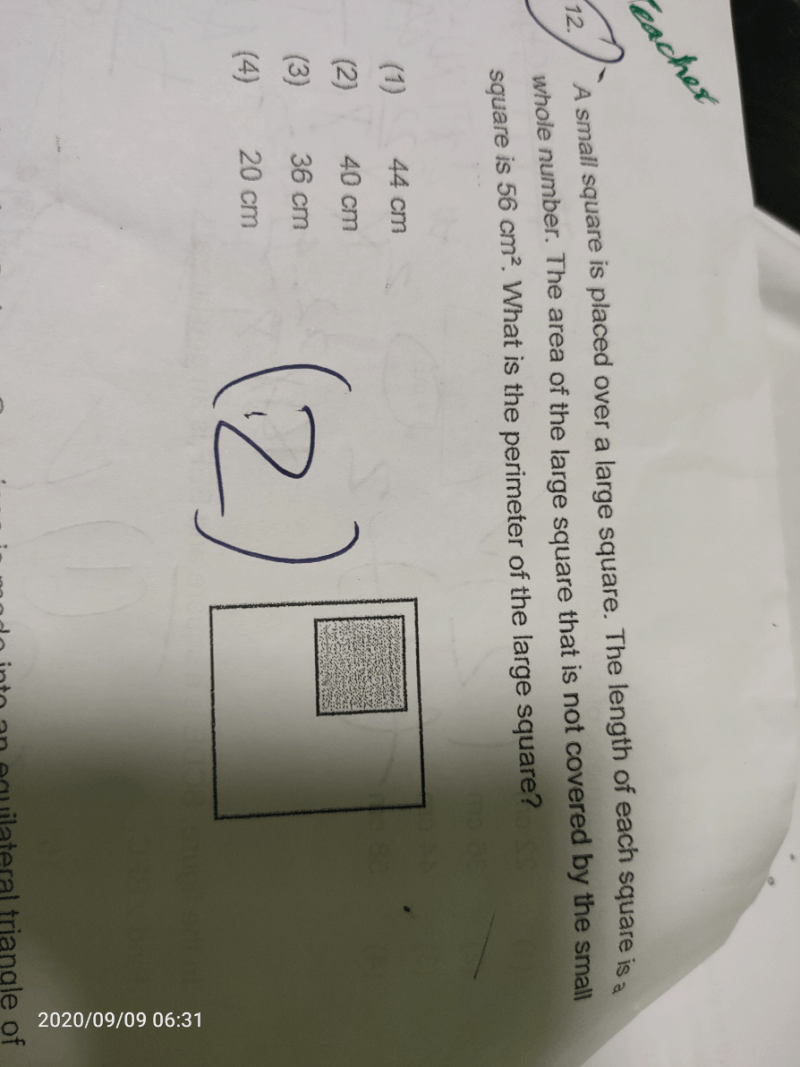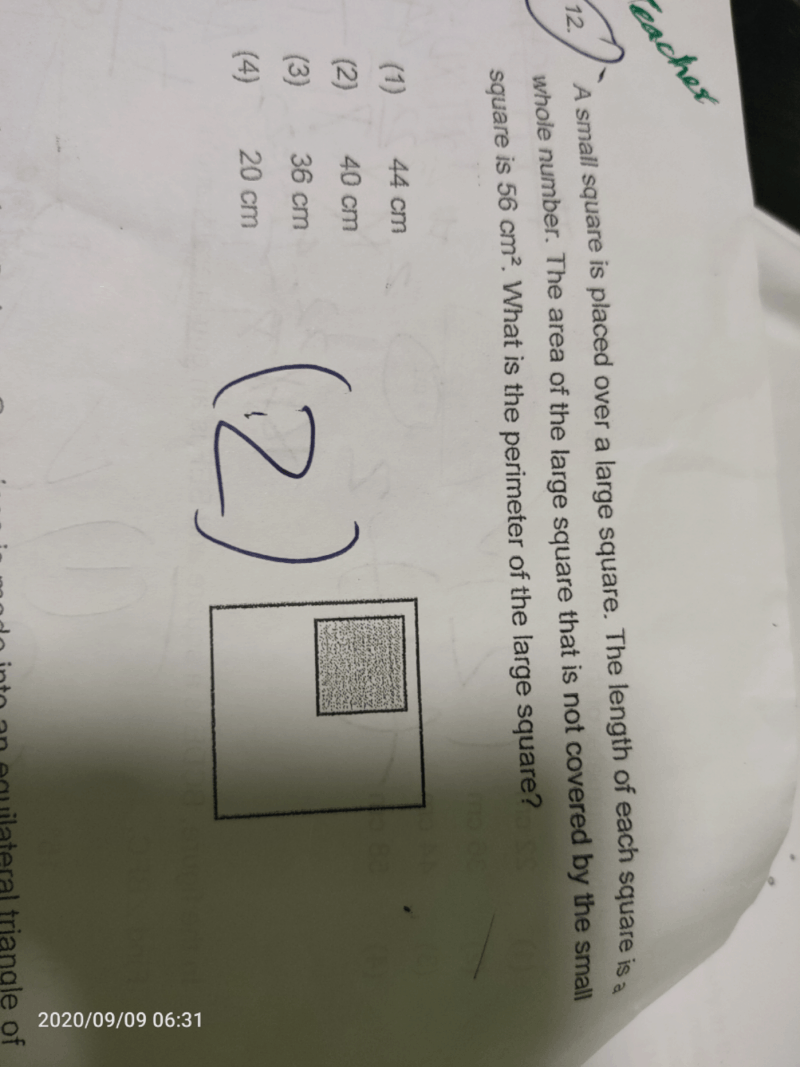# QuestionKnowing the area of the non shaded part is 56 cm², the area of the big square must be 8² cm² or more.

Taking the area of big square minus 56 cm², the answer must be able to be square rooted to a whole number as stated in the question.

 Length of big square (cm) Area of big square (cm²) Area of small square (area of big square – 56) Length of small square (cm) 8 64 8 2.8284 9 81 25 5 10 100 44 6.6332 11 121 65 8.062

Therefore, length of big square is 9 cm.

Answer: 9 × 4 = 36 cm

0 Replies 0 LikesThis one is very simple. We just need to take every answer and divide by 4. With this, we know how long one side of the large square is. For 1, it will be 11cm; 2, it will be 10cm; 3, it will be 9 cm and 4, it will be 5cm. We now have to square root all of these numbers. The results are that only 9cm is able to be square rooted into a whole number, so from that we get our answer; 3.

2 Replies 0 Likes

Thank you for the solution , but sorry i cant understand why we need to do this method ? also we are not using the info 56 cm2 given in the question , Could you please explain

0 Replies 0 Likes